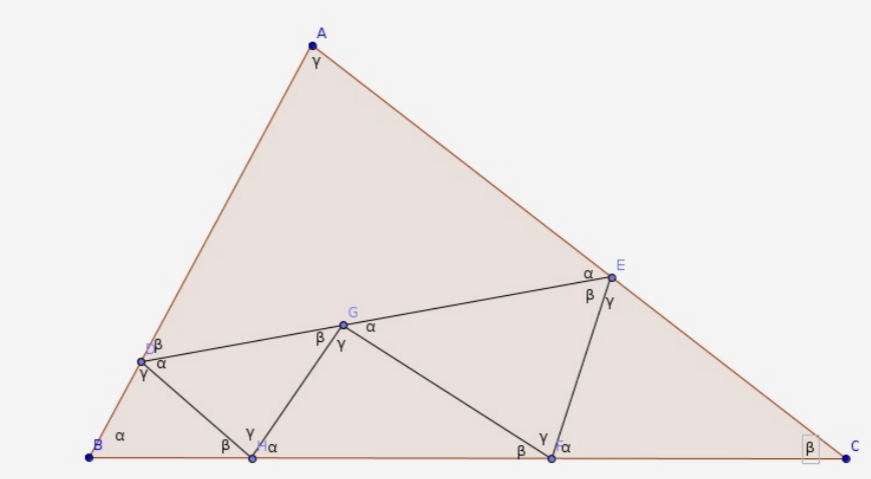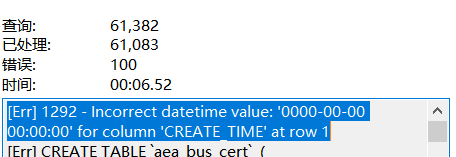2019字节跳动ACM程序设计冬令营题解#day1

###【E√，F√，G√，L√】 　　水题/老套路题。 ###【B，H，I】 　　待补。

###【A√】 　　题意：有 $k(\leq 1000)$ 个选手和 $N(\leq 1000)$ 个评委。每个评委给出一个长度为 $k$ 的排列，表示对每个选手的喜欢程度。选手的最终名次是这么决定的：从 $1$ 号评委开始，每次当前评委选取最厌恶的（且名次待定的）选手，将其设为名次待定选手中的最后一名。如果 $n<k$，评委循环决策，直到确定了所有选手的名次。现在你是 $v$ 号评委，只有你还未给出排列。你关注 $k$ 号选手。现在你能任意设定排列，求 $k$ 号选手最终排名最好能是多少。 　　题解：先假设没有评委 $v$ 模拟一遍，确定出依次被确定出的选手编号（轮到第 $v$ 号评委时用 $o$ 表示）： $xxxxoxxxxoxxyxoxx\dots$。设 $y$ 表示 $k$ 的位置。现在我们要找一些选手将它们填到 $o$ 里，使得 $y$ 的位置尽量靠后。首先，优先放 $y$ 之后的所有选手，因为这样 $y$ 的排名不发生变化。如果还有空格需要填 $y$，此时我们会发现，无论把哪个数字提前，$y$ 的位置必然会前进。而如果我们每次把 $y$ 之前的那个数提上去，$y$ 的位置必然前进 $1$ ——即这必然是最优解。所以确定 $v$ 号评委排列时，我们只需先把 $x$ 后面的数字塞进去，随后把 $x$ 前面的数字逆序塞进去。

###【C√】 　　题意：给出一个长度小于 $5000$ 的字符串。将其所有子串排序并连接。有 $M(\leq 5000)$ 个询问，每次问新串第 $k$ 位的字符是什么。 　　题解：构出后缀自动机直接遍历的复杂度是 $O(N^2 \sum)$ 的，不太优美。 　　观察 $height$ 数组。假设我们在考虑 $l \sim r$ 这些排名的后缀。设 $L=min(ht[l+1] \dots h[r])$，容易发现，长度为 $1,2,\dots,L$ 的前缀必然依次排布在新串开头，且各有 $r-l+1$ 个。对于长度更长的子串，我们可以把$(l,r)$ 分成两半，这两半字典序严格递增。现在问题独立，先递归前一半再递归后一半。单次询问复杂度 $O(|S|)$，总复杂度 $O(|S|^2+M|S|)$。

###【D√】 　　题意：给出一个长度小于 $3000$ 的字符串，求有多少种划分方案，使得每一段字典序都是严格递增的。 　　题解：有个经典操作是，设 $diff_{i,j}$ 表示后缀 $i$ 和后缀 $j$ 的第一个不同位置，这个可以递推预处理出。倒着dp，设 $f_{i,j}$ 表示后缀 $i$ 的划分里，第一段是 $S_{i \sim j}$ 的方案数。转移时枚举 $k$，把合法的 $f_{j+1,j}$ 累加进答案。显然 $k$ 是有单调性的，可直接根据 $diff_{i,j}$ 计算出来，再套个前缀和优化即可。

###【J√】 　　题意：给出两棵 $2N-2(N \leq 1000)$ 个点的树，保证每个点度数是 $1$ 或 $3$，且叶节点编号为 $1 \sim N$。问有多少对四元组 $(A,B;C,D)$，满足 $A,B,C,D$ 是叶子，且第一棵树上 $A \sim B$，$C \sim D$ 的有交性和第二棵树上相反。 　　题解：转化成：求在两棵树上都不交的四元组个数。考虑枚举 $(A,B)$ 的 $LCA$ 统计个数。因为我们在无根树上考虑问题，要拓展这个 $LCA$ 的“定义”。严格地来说，对于一组合法的 $(A,B,C,D)$，定义 $x$ 为 $A \sim B$ 路径上里路径 $C \sim D$ 最近的点。我们每次枚举第一棵树上的 $x$ 和第二棵树上的 $x$ 并统计答案。容易发现，每个合法四元组会被统计两次。 　　每次枚举度数为 $3$ 的点。其中两个度分别放 $A$ 和 $B$，另一侧放 $(C,D)$（只需统计点的个数 $t$，方案数就是 $C_t^2$）。我们可以借助有根树的 $DFS$ 序快速维护答案。因为有两棵树，可行点数是它们的交。这是一个经典问题，对于一个点 $x$，假设它在两棵树上的 DFS 序是 $(u_x,v_x)$，将其看做一个点，每次就是矩形询问。因为是离线的，我们可以二维前缀和预处理，就支持 $O(1)$ 询问啦。 　　还有一种直接 DP 的做法：我们枚举第一棵树 $(A,B)$ 的 LCA。将第一个分叉的所有叶子在第二棵树的对应位置标为 $1$，第二个分叉标为 $2$，第三个分叉标为 $3$。对于第二棵树里的所有从 $1$ 到 $2$ 的路径，它将该树化成了若干个连通块，我们要求每个联通块 $C_X^2$ 的和。这可以通过比较麻烦的树形 DP $O(N)$ 求出来。总复杂度也是 $O(N^2)$。

###【K√】 　　题意：有一个 $N \times N(N \leq 300)$ 的数字矩阵。求一个子矩阵，使得 “数字和” 比上 “周长” 最大。 　　题解：直接做是 $N^3 log V$ 的：枚举上下边界后分数规划。有一个小 trick是：每次做到一组上下边界时，先去 check 一下当前答案，如果不合法直接退出二分过程。这个做法的理论复杂度是 $O(N^3+N^2 \log V)$ 的。 　　上述证明可以抽象成这么一个问题。我们有 $N^2$ 个箱子，每个箱子可以 $O(N \log V)$ 确定它的解，或者 $O(N)$ 验证一组解可不可行。现在要求所有箱子解的最大值。考虑一种做法：每次随机选择一个箱子确定它的最优解 $X$。然后遍历所有箱子 $O(N)$ check，如果 $X$ 不是该箱子的解就扔掉这个箱子。对于剩下的箱子继续这么做。这样的复杂度是 $T(M)=N \log V+MN+T(x)$，其中 $x$ 是 $0 \sim M$ 里随机的。事实上，这个复杂度等于 $T(M)=N \log V+MN+T(\frac{N}{2})$。

#day2

###【A，F，H，I】 　　不补了。 ###【B√】 　　题意：有 $N(\leq 100000)$ 个物品，每个物品有两维 $(p_i,q_i)$。现在最多可以用两次 $split$ 操作。每次选择一个物品和一个常数 $p(0 < p < 1)$，将物品按 $p$ 等比例拆成两个。操作完后，要将物品分成两堆，每堆两维的和都彼此相同。 　　题解：先考虑一维怎么做。将物品看成线段，整齐地铺在数轴上。我们在 $\frac{sumL}{2}$ 处切一刀，切到的那个物品需要被 $split$ 一次。 　　两维需要一点小技巧。将每个物品看做一个底边为 $p_i$，高为 $\frac{q_i}{p_i}$ 的长方形。我们需要在 $X$ 和 $X+\frac{\sum p_i}{2}$ 处切，使得中间那一块长方形的面积和是总面积的一半。如果我们按照 $\frac{q_i}{p_i}$ 将长方形排序，那么切出来的面积和切的位置 $X$ 是正相关的。二分即可，复杂度 $O(N \log V)$；也可以单调地扫描过去，每次解一个二次方程，复杂度 $O(N \log N)$。

###【C√】 　　题意：考虑正 $N(\leq 5 \times 10^5)$ 边形上的 $N$ 个点。将它们构成一个边权只有 $0/1$ 的完全图。读入 $M$ 条边，剩下的所有边的颜色是根据一个参入读入的函数构造的。现在要构造一个生成树，要求每条边颜色必须相同，且这些边在正 $N$ 边形内不能相交。 　　题解：最后一个限制很难，反而在暗示，存在一种很简单的构造方法。我们先考虑 $N$ 条环边，如果颜色全一样，显然我们直接得到了一组解（去掉任意一条边即可）。否则，必然存在一个点 $x$，它左右的边颜色不同。假设它相邻的两个点是 $(u,v)$，我们先删掉 $x$，连上 $(u,v)$，递归地构造出 $N-1$ 个点的生成树。最后根据生成树颜色的黑白性，再连上和 $x$ 相连的边即可。递归到剩下 $2$ 个点时必然合法。用 $map$ 简单维护，总复杂度 $O(N \log N)$。

###【D√】 　　题意：给出一个 $N$ 个点的简单多边形。对于一个定点 $(x0,y0$，随机枚举一条穿过它的直线。求与多边形交的长度和的期望。 　　题解：有一个令人惊讶的性质是：就像有向线段计算面积一样，计算长度和也是可以每条有向边独立的。对于每条有向边，积分式是形如 $\int \frac{1}{cos(\theta)}$ 或是 $\int \frac{1}{sin(\theta)}$。上下同乘一个分母即可化为多项式积分。总复杂度 $O(N)$。

###【E√】 　　题意：给出一个字符串集合 $S_1,\dots,S_n$。要求合法的字符串集合 $T_j$ 的个数，使得每一个 $S_i$ 能且仅能找到一个 $j$ 使得 $T_j$ 是 $S_i$ 的前缀。同时规定了集合大小是 $M$。$(|S_i| \leq 200,N,M \leq 2000)$ 　　题解：建出 $S_i$ 的 $trie$ 树。相当于是要在树上选择 $M$ 个互不相同的点，使得每个叶子仅属于一个点的子树。设 $dp_{i,j}$ 表示决策完了 DFS序 第 $i$ 个叶子，且总共选了 $j$ 个点的方案数。如何选当前点？暴力枚举 $j$ 的祖先 $x$，如果$R_x=j$，那么可以从 $f_{L_x-1,j-1}$ 转移过来。注意到，暴力跳父亲直到不合法的总复杂度是 $O(VM)$ 的（$V$ 是总点数），因为一个点 $x$ 只会被 $R_x=j$ 的点跳到。因为 $V$ 可以很大，可以建出 $N$ 个点的虚树，将一条链上的点压成一个。

###【G√】 　　题意：在一棵 $N(\leq 10000)$ 个点的树上选择最多 $K(\leq 100)$ 条点不相交的路径，求路径上点数之和的最大值，并输出一种端点的方案。 　　题解：设 $f_{x,y,0/1}$ 表示 $x$ 子树里选了 $y$ 条链，且是否有一条链向上顶 的点数和的最大值。每次在子树里 $dp$ 时，是可能把两条路径并在一起的，所以转移时要设 $G_{i,y,0/1/2}$ 表示做到第 $i$ 个儿子，选了 $y$ 条链，且总共有 $0/1/2$ 三条支链向上顶的点数和的最大值。 　　在子树一个个dp过去的时候，再开一个数组记录一下对应方案即可还原。把 $y$ 限制成子树 $size$，复杂度就是 $O(NK)$ 了。题解的证明方法比较帅，分别考虑 $size$ 大于 $K$ 的和不大于 $K$ 的两部分，复杂度都是 $O(NK)$。

###【J√】

题意：定义 $F(N)$ 为：小于 $N$ 且与 $N$ 互质的数的和。设 $M=F(N)$，给出 $M$，求一个合法的 $N$。$1000$ 组数据，$M \leq 10^18$。 　　题解：$F(N)=\prod p_i^{2k_i-1} \times (p_i-1)$。如果我们能快速分解出 $M$，直接枚举选择了哪些 $p_i$，然后 $O(\log M)$ 确认即可。 　　直接跑 rho 太慢了。考虑先预处理出前 $10^6$ 的质数。对 $M$ 筛后，最多只会出现 $M'=P$ 或 $M'=p^2$ 或 $M'=PQ$ 三种形式。前两种都可以特判，终点考虑第三种。推理一下可知，$10^6<P<Q<10^9$，且$Q$ 这个质数必然出现在 $p_i$ 里，所以 $P$ 必然不出现。因为 $P|(Q-1)$，我们可以直接枚举 $\frac{Q-1}{P}$ 的值 check 即可，每次只要枚举到 $1000$。

###【K√】

题意：给出 $N(\leq 250)$ 个点，保证没有三点贡献。要在里面选出 $5$ 个点，$3$ 个构成三角形三个顶点，$2$ 个构成线段。要求线段和三角形严格不交，问有几种选法。

#day3

###【A，E，I】 　　简单题。

###【H】 　　待补。

###【F，J】 　　不补了。

###【B√】

题意：给出一个 $N(\leq 20)$ 个点的确定性自动机，边上是小写字母。从 $1$ 号点出发，每次随机找一条出边走过去，并把沿途的字母连接成字符串 $S$。再给出两个字符串 $(A,B) (|A| \leq 10,|B| \leq 50)$，任意时刻当 $A$ 是 $S$ 子串或 $B$ 是 $S$ 子序列时停止游走。问路径的期望长度，无穷大输出 $-1$。 　　题解：很清晰的高斯消元题。子序列的匹配具有单调性，所以可以按 $B$ 串分层进行高斯消元。每层共有 $N(|A|+1)$ 个点，依据 $fail$ 数组建立方程。注意最好先判一下无穷大的情况，包括：①存在一条路径连向下一层或本层的无穷大。②本层一个封闭的圈，不连向任何已经确定的答案。

###【C√】

题意：给出平面上的 $N(\leq 2000)$ 个点，求一个面积最小的三角形。 　　题解：先枚举三角形的最低点，将它上方的点极角排序。注意，极角序相邻的点不一定就是答案：枚举了其中一个向量后，另一个点必须是距离离它最小的。对于极角序相邻的两个 $(x,y)$，做一条从原点开始与其连线平行的向量 $\gamma$。观察到，$x$ 到 $\gamma$ 一侧的距离都优于 $y$，而 $y$ 在另一侧比较优。所以我们可以按极角序压入一些点，维护一个凸壳表示可能成为答案的点集。随着枚举的向量变化，可能会弹出凸壳顶。

###【D√】

题意：给出一张 $N(\leq 1000)$ 个点，$M(\leq 100000)$ 条边的无向图，保证每一个点度数都一样且是偶数。现在要删掉若干边，使得剩下的图里每个点的度数都是 $2$。 　　题解：考虑网络流模型：左右两排都是 $N$ 个点，与起点终点的限制流量都是 $1$；原图的一条边 $(u,v)$ 在正反连两次。如果满流，每个点都有一个唯一的出点，我们可以根据这个连出若干个环，问题也就解决了。 　　实际上还有一个小问题：这样可能会连成二元环，而二元环是不被允许的。所以我们希望原图的每条边只在网络流里被连一次，即给边定向，使得有解性不变。有一种很优美的定向方法：在原图中做一遍欧拉回路，每条边被经过的方向即为它的方向。 　　现在我们来证明，这种做法必然能找到解。欧拉回路完后，每个点正好有 $k$ 条出边和 $k$ 条入边。在网络流的二分图里，考虑左侧的一个集合 $X$，它们的出度和是 $k|X|$。因为右侧每个点入度也是 $k$，所以与 $X$ 关联的右侧点至少有 $k$ 个。由霍尔定理，这个二分图存在完备匹配。

###【G√】

题意：将俄罗斯方块（包含所有旋转型）填入 $4 \times N$ 的格子中。求能填满格子的方案数。$N \leq 10^9$。 　　题解：注意俄罗斯方块及其旋转型 其实就是所有四连通的网格。 　　一列一列填过去，最多只和前三列有关。可以状压前 $4 \times 3$ 的格子信息进行 dp。 　　有一种策略是直接暴力 $dp$ 1000 项，得到的数列必然是线性递推数列，找寻一下规律即可。 　　也可以对上限是 $2^{12}$ 种状态进行压缩（因为很多状态可能永远也填不满了）。比赛时直接加了些无脑剪枝，比如 "任意一个白点到右侧那一列的最短路不能超过 $4$"，“假设第三列的白色方块数量是 $k$，则左三列白色方块不能超过 $3k$”，"如果存在一个 $1 \times 4$ 的白条，先假设填上，若之后的状态不行则它也不行"。这样状态只有 $360$ 种左右了。再应用对称性可以缩到 $180$ 种，预处理转移直接矩乘。

###【K√】

题意：$2N$ 个点排成一个环。要将每两人匹配成一个组，使得在环上有边的组正好有 $K$ 组。$1 \times K \times N \leq 10000$ 　　题解：

#day4

###【A，E，I，J，K】 　　简单题 / 码农题。

###【B】

题意：求无穷级数 $\sum \frac{\ln n}{n^s}$ 的值。和标准值的绝对误差不能超过 $1e-6$，$1.0001 \leq s \leq 20$ , $s$ 四位小数。 　　题解：待补。

###【C√】 　　题意：给出一个字符串 $S(|S| \leq 10^5)$。有 $Q(\leq 3 \times 10^5)$ 个询问，每次给出 $(l,r)$，问 $l \sim r$ 之间有多少本质不同的子串。 　　题解：如果用 $manacher$ 求出每个位置的回文半径 $p_i$，那么对于一个询问 $(l,r)$，答案就是 $\sum_{i=l}^r \min(p_i,i-l+1,r-i+1)$。比赛时我想的是，每次枚举一个位置 $i$，去维护它对所有询问 $(l,r)$ 的贡献。$min$ 里面有 $3$ 个量不太好直接维护。假设我们维护好了$(l,r)$ 的答案，如果边界变动一格，我们可以用主席树在 $O(\log N)$ 的时间内求出增量（比如 $l$ 减一，就是询问 $\sum_{i=l}^{(l+r)/2} [p_i<l]$。那么我们可以分块，先用差分数组在 $O(N \sqrt N)$ 时间内维护出 $f_{b,r}$ ，表示第 $l$ 个块的开头到位置 $r$ 的答案。每次询问时移动 $l$ 求增量，询问复杂度 $O(Q \sqrt N\log N)$。但这样是过不了的。发现每次移动的询问是彼此独立的，可以先离线下来（为了防止空间不够，可以每隔几百万离线求一次），用桶排+树状数组快速求出答案，几乎可以把原来的主席树的 $O(\log N)$ 的常数给拿掉。 　　比赛中智商降了好多……反过来考虑，固定一个 $(l,r)$，求所有 $p$ 对它的贡献。 根据 $mid$ 划成两半，三维 $min$ 就直接变成了二维 $min$……然后离线+线段树或者直接主席树就在 $(N \log N)$ 时间内轻松解决了……

###【D】 　　题意：给出平面直角坐标系里的三个整点表示一个三角形。现在要将其划成若干个小三角形，使得每个小三角形都和原图形相似，且大小两两不同。 　　题解：只有正三角形是无解（但是三个整点表示不出正三角形）。在大多数情况都可以用以下Case解决：### 【F】

题意：有一个 $N \times M (N,M \leq 1000)$ 的网格，$(x,y)$ 是障碍。用大小为 $3$ 的 $L$ 型骨牌去覆盖剩下的格子，打印一种方案或输出无解。 　　题解：待补。

### 【G√】

题意：图上有 $N$ 个点，起初没有边。有 $M$ 个操作，每次加入一条边 $(u,v)$ 或者删除一条边。每次操作完后询问桥的个数。 　　题解：经典的动态桥边计数问题， claris的题解 。设 $solve(l,r,n,m)$ 为考虑时间在 $[l,r]$ 的修改操作，作用范围是 $n$ 个点，$m$ 条边的图。设 $E$ 表示 $[l,r]$ 涉及到的边集，$V$ 表示它们两端涉及到的点集。先将 $M-E$ 跑一遍 $tarjan$，将边双缩点（边双内部的边永远不会是桥）。在剩下的树（森林）中，做出 $V$ 的虚树。不在虚树上的边永远都是桥，直接加入答案即可。将剩下的虚树做一张新图，递归左右两个子问题（有个细节是，虚树上的一条边要额外记录对应了原图的几条边，一旦它成为答案，这些边都是桥）。容易发现，每一层做完后，新图的点数和边数都是 $O(r-l)$。不计并查集复杂度，总复杂度为 $M \log M$。 　　还有一种 $M \log M \log N$ 的 LCT 做法。将边插入时间线段树，现在只剩下加边了。用 LCT 维护当前森林，如果进来一条边 $(u,v)$ 所在的连通块已经连通，就在 $u \sim v$ 的路径上区间加 $1$。显然，桥的个数即为权值为 $0$ 的边的条数，这个可通过在 LCT 的 Splay 上维护子树最小值以及子树最小值个数来实现。注意，每次加入一条边后，只需考虑答案的增量，所以只有链上询问最小值个数，不需要带虚儿子和的 LCT。时间线段树里的回退也能很方便地实现。

### 【H√】

题意：有一棵 $N(\leq 10^5)$ 个点的树，每条边有边权 $(b_i,c_i)(\leq 10^9)$。对于一条边 $i$，定义 $d_i$ 为：考虑根到它的其它所有 $b_j<b_i$ 的边 $j$。如果不存在这样的 $j$，则 $d_i=c_i$；否则 $d_i=median(d_j)+1$，$median$ 表示符合要求的边的 $d_j$ 的中位数。 　　题解：在树上DFS。套一个树状数组来满足 $b_i$ 这一维的限制，每个 bit 对应一棵动态开点的权值线段树（用来维护 $d_i$）。查询时，在符合要求的 $log$ 个权值线段树里一起走。时间和空间复杂度都是 $O(N \log^2 N)$。

### 【I√】

题意：给出 $N(\leq 1111)$ 个不经过原点的平面。从原点开始选择一条射线射出去，问最多能射到多少平面。 　　题解：转化的问题是：给出 $N$ 个三维向量。求一个向量 $(x,y,z)$，使得与它们分别点积后 大于 $0$ 的情况数最多。 　　我们考虑以这个向量为法向量的平面。由调整法，存在一个最优解，使得这个平面过某个给定的向量。那么我们 $O(N)$ 枚举每一个向量，以这个向量为一条边，旋转一周，去寻找最优秀的平面。这时可以转化为，在二维坐标里旋转一周，找一侧点最多的直线。极角排序即可，复杂度 $O(N^2 \log N)$。blmius
2年前
MySQL:[Err] 1292 - Incorrect datetime value: ‘0000-00-00 00:00:00‘ for column ‘CREATE_TIME‘ at row 1Jacquelyn38
2年前
2020年前端实用代码段，为你的工作保驾护航Easter79
2年前
swap空间的增减方法
（1）增大swap空间去激活swap交换区：swapoff v /dev/vg00/lvswap扩展交换lv：lvextend L 10G /dev/vg00/lvswap重新生成swap交换区：mkswap /dev/vg00/lvswap激活新生成的交换区：swapon v /dev/vg00/lvswaphelloworld_34035044
1年前

​在我们用皕杰报表工具设计填报报表时，如何在新增行里自动增加id呢？能新增整数排序id吗？目前可以在新增行里自动增加id，但只能用uuid函数增加UUID编码，不能新增整数排序id。uuid函数说明：获取一个UUID，可以在填报表中用来创建数据ID语法：uuid()或uuid(sep)参数说明：sep布尔值，生成的uuid中是否包含分隔符''，缺省为Stella981
2年前
MSTP+VRRP+OSPF+双出口Stella981
2年前
KVM调整cpu和内存Stella981
2年前
Android So动态加载 优雅实现与原理分析Stella981
2年前
SAP成都研究院DevOps那些事Wesley13
2年前
00：Java简单了解Wesley13
2年前
MySQL部分从库上面因为大量的临时表tmp_table造成慢查询Wesley13
Lv1

1.2w

18

1.7k